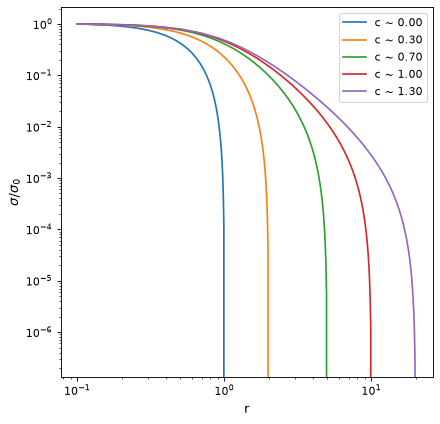# KingProjectedAnalytic1D¶

class astropy.modeling.functional_models.KingProjectedAnalytic1D(amplitude=1, r_core=1, r_tide=2, **kwargs)[source]

Projected (surface density) analytic King Model.

Parameters
amplitudefloat

Amplitude or scaling factor.

r_corefloat

Core radius (f(r_c) ~ 0.5 f_0)

r_tidefloat

Other Parameters
fixeda dict, optional

A dictionary {parameter_name: boolean} of parameters to not be varied during fitting. True means the parameter is held fixed. Alternatively the fixed property of a parameter may be used.

tieddict, optional

A dictionary {parameter_name: callable} of parameters which are linked to some other parameter. The dictionary values are callables providing the linking relationship. Alternatively the tied property of a parameter may be used.

boundsdict, optional

A dictionary {parameter_name: value} of lower and upper bounds of parameters. Keys are parameter names. Values are a list or a tuple of length 2 giving the desired range for the parameter. Alternatively, the min and max properties of a parameter may be used.

eqconslist, optional

A list of functions of length n such that eqcons[j](x0,*args) == 0.0 in a successfully optimized problem.

ineqconslist, optional

A list of functions of length n such that ieqcons[j](x0,*args) >= 0.0 is a successfully optimized problem.

Notes

This model approximates a King model with an analytic function. The derivation of this equation can be found in King ‘62 (equation 14). This is just an approximation of the full model and the parameters derived from this model should be taken with caution. It usually works for models with a concentration (c = log10(r_t/r_c) parameter < 2.

Model formula:

$f(x) = A r_c^2 \left(\frac{1}{\sqrt{(x^2 + r_c^2)}} - \frac{1}{\sqrt{(r_t^2 + r_c^2)}}\right)^2$

References

1

Examples

import numpy as np
from astropy.modeling.models import KingProjectedAnalytic1D
import matplotlib.pyplot as plt

plt.figure()
rt_list = [1, 2, 5, 10, 20]
for rt in rt_list:
r = np.linspace(0.1, rt, 100)

mod = KingProjectedAnalytic1D(amplitude = 1, r_core = 1., r_tide = rt)
sig = mod(r)

plt.loglog(r, sig/sig, label=f"c ~ {mod.concentration:0.2f}")

plt.xlabel("r")
plt.ylabel(r"$\sigma/\sigma_0$")
plt.legend()
plt.show()


(png, svg, pdf)Attributes Summary

 amplitude concentration Concentration parameter of the king model input_units This property is used to indicate what units or sets of units the evaluate method expects, and returns a dictionary mapping inputs to units (or None if any units are accepted). param_names Names of the parameters that describe models of this type. r_core r_tide

Methods Summary

 evaluate(x, amplitude, r_core, r_tide) Analytic King model function. fit_deriv(x, amplitude, r_core, r_tide) Analytic King model function derivatives.

Attributes Documentation

amplitude = Parameter('amplitude', value=1.0, bounds=(1.1754943508222875e-38, None))
concentration

Concentration parameter of the king model

input_units
param_names = ('amplitude', 'r_core', 'r_tide')

Names of the parameters that describe models of this type.

The parameters in this tuple are in the same order they should be passed in when initializing a model of a specific type. Some types of models, such as polynomial models, have a different number of parameters depending on some other property of the model, such as the degree.

When defining a custom model class the value of this attribute is automatically set by the Parameter attributes defined in the class body.

r_core = Parameter('r_core', value=1.0, bounds=(1.1754943508222875e-38, None))
r_tide = Parameter('r_tide', value=2.0, bounds=(1.1754943508222875e-38, None))

Methods Documentation

static evaluate(x, amplitude, r_core, r_tide)[source]

Analytic King model function.

static fit_deriv(x, amplitude, r_core, r_tide)[source]

Analytic King model function derivatives.